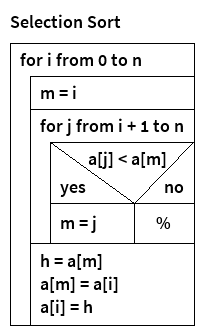# Nassi-Shneiderman Diagram Generator 1.0

I released the first version of my Nassi-Shneiderman Diagram Generator!

A Nassi-Shneiderman diagram is a graphical representation of a computer program (read more on Wikipedia).

This Java program converts xml descriptions of these diagrams into image files. Here is an example:This image was generated from the following xml document:

```<diagram title="Selection Sort">
<loop condition="for i from 0 to n">
<block>m = i</block>
<loop condition="for j from i + 1 to n">
<branch condition="a[j] \l a[m]">
<left label="yes">
<block>m = j</block>
</left>
<right label="no" />
</branch>
</loop>
<block>
h = a[m]
a[m] = a[i]
a[i] = h
</block>
</loop>
</diagram>
```